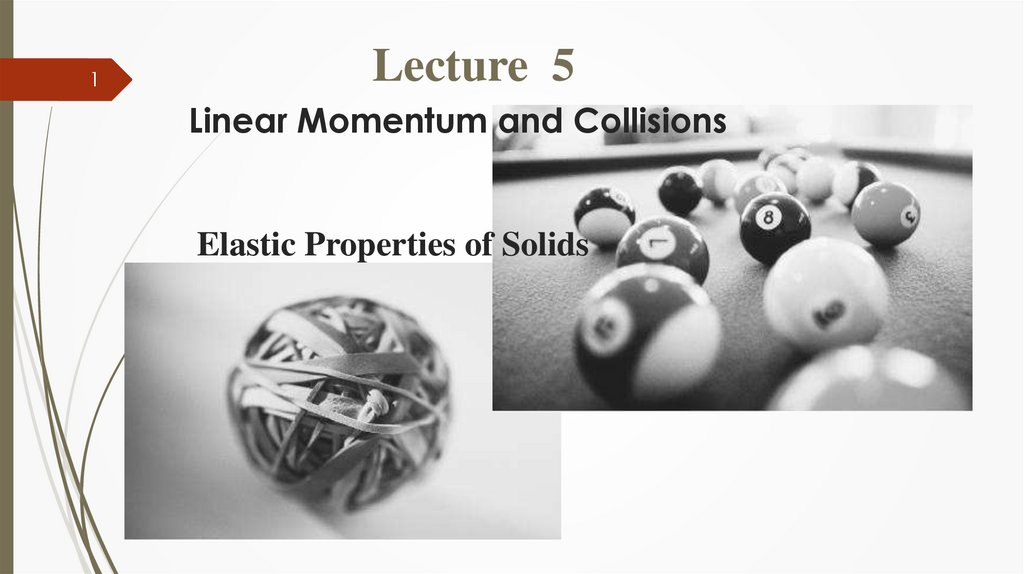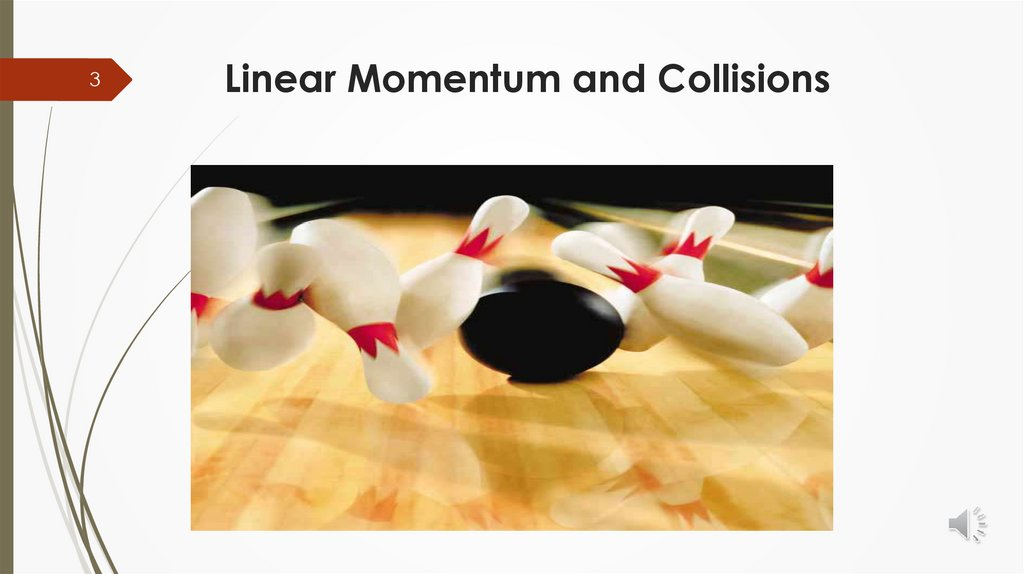# Linear Momentum and Collisions Elastic Properties of Solids. Lecture 5

## 1.

1
Lecture 5
Linear Momentum and Collisions
Elastic Properties of Solids

## 2.

https://youtu.be/Ug97oHED854

## 3.

3
Linear Momentum and Collisions

## 4.

Linear Momentum
4
Is
defined to be equal to the mass of an
object times its velocity.
p = mv
Momentum is a vector quantity.
The vector for linear momentum points in the
same p direction as the velocity v.
S.I unit of momentum : Kg . m/s

## 5.

5
Linear Momentum
The relationship between m & v
Huge ship moving at a small velocity
P = Mv
High velocity bullet
P = mv

## 6.

Linear Momentum
The relationship between m & v
6
Example :
A 10,000 kg truck moving at 2 m/s has a linear momentum
of 20,000 kg.m/s ,while a 80 kg bicyclist moving at 2 m/s
has a linear momentum of 160 kg m/s.
The truck has a much larger linear momentum even though
both are moving at the same velocity.
It is easier to bring the bicyclist to a stop than it is to bring
the truck to a stop.
Similarly, it is easier to stop a bicyclist moving at 2 m/s
than a bicyclist moving at 5 m/s.

## 7.

7
Collision & linear
momentum
The types of collision
• Elastic collision
• Inelastic collision
Momentum of the system is conserved
in all collisions, but kinetic energy of the
system is conserved only in elastic collisions.

## 8.

In elastic collision: both momentum and kinetic energy of the system are conserved.
In inelastic collision: momentum is conserved BUT kinetic energy is not conserved.
Ref. https://www.physicstutorials.org/pt/44-Collisions

## 9.

9
Collision & linear
momentum
Another way to compare linear momentum
is to consider a collision.
If a boy is running at you at full steam and hits you,
you'll probably be knocked down but will still be okay.
However, if a truck is coming at you at the same speed
and hits you, you will be hurt badly.
In this example, the boy has much less linear momentum
than the larger truck.

## 10.

10
Conservation of momentum
Whenever two or more particles
in an isolated system (frictionless, no loss of
energy) interact,
the total momentum of the system remains
constant.
total linear momentum before = total linear momentum after

## 11.

Linear Momentum and
Its Conservation
11
If an object's velocity is changing with time,
its linear momentum is changing. We have
dp/dt = d(mv)/dt
If the mass of the object is constant then
dp/dt = mdv/dt = ma
We write
dp/dt = F = ma
* If the force F = o , That means linear momentum is constant.
This is a more general statement of Newton's second law
which also holds for objects whose mass is not constant.

## 12.

12
Example : The Archer
Let us consider the situation
proposed at the beginning of this
section. A 60-kg archer stands at
rest on frictionless ice and fires a
0.50-kg arrow horizontally at 50 m/s.
With what velocity does the archer
move across the ice after firing the
arrow?

## 13.

13
Example : Conservation of
momentum

## 14.

Linear Momentum and
Its Conservation
14
Quick Quiz 9.1 Two objects have equal kinetic
energies. How do the magnitudes of their momentum
compare?
(a) p1 > p2
(c) p1 < p2
(b) p1 = p2
(d) not enough information to tell.

## 15.

15
Linear Momentum and
Its Conservation

## 17.

Elastic Properties of Solids
All objects are deformable
when external forces act on them.
That is,
it is possible to change the shape or the size
(or both) of an object by applying external forces.

## 18.

Elastic Properties of Solids
Stress
is the external force acting on an object per unit
cross-sectional area.
Stress = F \ A
is a quantity that is proportional to the force
causing a deformation.
The unit of Stress in SI system is ……….
The result of a stress is strain, which is
a measure of the degree of deformation.

## 19.

Elastic Properties of Solids
The result of a stress is strain, which is
a measure of the degree of deformation.
Strain is proportional to stress. ( Strain Stress )
The constant of proportionality ( ) is called
the elastic modulus.

## 20.

Elastic Properties of Solids
The types of an elastic modulus :
1.
Young’s modulus, which measures the
resistance of a solid to a change in its length.
2.
Shear modulus, which measures
the resistance to motion of the planes
within a solid parallel to each other.
3., which measures the resistance of solids or fluids
to changes in their volume.

## 21.

Elastic Properties of Solids
The elastic modulus:
Is defined as the ratio of the stress to
the resulting: strain.
Elastic modulus = stress / strain
The elastic modulus relates what is done to a solid object (a force is
applied) to how that object responds (it deforms to some extent).

## 22.

Elastic Modulus
h
Young’s modulus is defined as :
Tensile stress: the ratio of the magnitude of the
external force F to the cross-sectional area A.
Tensile strain: in this case the ratio of the change
in length ΔL to the original length Li.

## 23.

Elastic Modulus
h
Young’s modulus is defined as :
The unit of young ‘s modulus is the ratio of
that for force to that for area. ( N \ m2 )

## 24.

h
The elastic limit
The elastic limit of
a substance is defined
as the maximum stress
that can be applied to
the substance before it
becomes permanently
deformed and does not
length.

## 25.

Another type of deformation occurs when an object is subjected
to a force parallel to one
of its faces while the opposite face is held fixed by another
force.

## 26.

Shear stress: ratio of the tangential force to the area A of the face being sheared.
Shear strain: ratio Δx/h, where Δx is the horizontal distance that the sheared face
moves and h is the height of the object.
The unit of shear modulus is
the ratio of that for force to that for area.

## 27.

Volume stress: ratio of magnitude of total force F exerted
on a surface to the area A of the surface.
P = F/A is called pressure. If it changes by an amount ΔP
= ΔF/A, then the object will experience a volume change
ΔV.
Volume strain: is equal to the change in volume ΔV
divided by the initial volume Vi.

## 28.

The unit of bulk modulus is
the ratio of that for force to that for
area.
Note that both solids and liquids have
a bulk modulus. However, no shear
modulus and no Young’s modulus are
given for fluids. ( Why )

## 29.

Because a liquid does not sustain a shearing
stress or a tensile stress.
If a shearing force or a tensile force is applied to
a liquid, the liquid simply flows in response.

## 30.

Homework
1. Can a bullet have the same momentum as a truck?
Explain.
2- Two materials, A and B, are used to make cables of
identical cross section and length, to lift identical loads.
If A has a greater Young’s Modulus than B, which cable
will stretch the most when loaded? Explain why.